Next: The discrete Fourier transform Up: Computer Vision IT412 Previous: Lecture 4

Subsections

# Fourier transform theory

So far we have been processing images by looking at the grey level at each point in the image. These methods are known as spatial methods.

However, there are many ways of transforming image data into alternative representations that are more amenable for certain types of analysis. The most common image transform takes spatial data and transforms it into frequency data. This is done using the Fourier transform.

The Fourier transform is simply a method of expressing a function (which is a point in some infinite dimensional vector space of functions) in terms of the sum of its projections onto a set of basis functions. Since an image is only defined on a closed and bounded domain (the image window), we can assume that the image is defined as being zero outside this window. In other words, we can assume that the image function is integrable over the real line.

To see how the Fourier transform works, we will begin with a one-dimensional signal and consider a simple step function. This is equivalent to taking a horizontal slice through an image that is black on its left half and white on its right half, as shown in figure 1.

Now, a step function (or a square wave form) can be represented as a sum of sine waves of frequency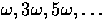, where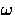is the frequency of the square wave, and we recall that frequency = 1/wavelength. Normally, frequency refers to the rate of repetitions per unit time, that is, the number of cycles per second (Hertz). In images we are concerned with spatial frequency, that is, the rate at which brightness in the image varies across the image, or varies with viewing angle. Figure 2 shows the sum of the first few terms in a sine wave decomposition of a square wave. This sum converges to the square wave as the number of terms tends to infinity.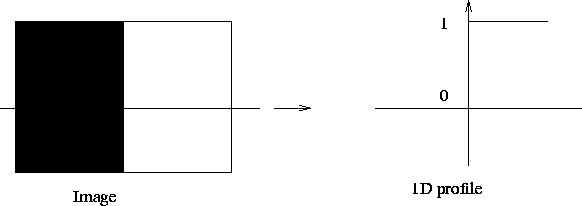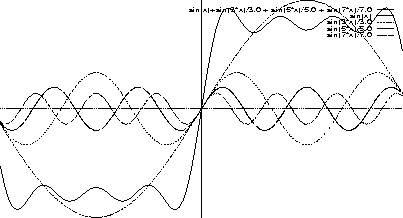From the decomposition of the signal into varying sinusoidal components we can construct a diagram displaying the amplitudes of all the sinusoids for all the frequencies. A graph of such a diagram is given in figure 3 below for the square wave.

Note that we have to consider negative frequencies (whatever that might actually mean) so the sinusoidal component of frequency f and amplitude A1 has to be split into two components of amplitude A1/2 at the frequencies +f and -f. A graph of the amplitude of the Fourier components is known as the spectrum of the wave form.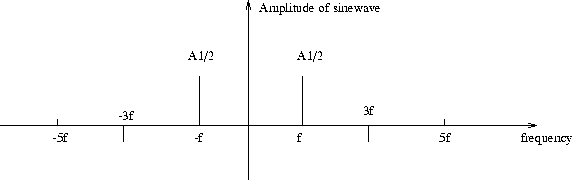So what does the Fourier transform really mean? When we calculate the Fourier transform of an image, we treat the intensity signal across the image as a function, not just an array of values. The Fourier transform describes a way of decomposing a function into a sum of orthogonal basis functions in just the same way as we decompose a point in Euclidean space into the sum of its basis vector components.

For example, a vector v in 3-space is described in terms of 3 orthogonal unit vectors i, j and k, and we can write v as the sum of its projections onto these 3 basis vectors:Given the vector v, we can calculate the components of v in each of the i, j, and k directions by calculating the dot product (or inner product or projection) of v and each of these basis vectors. ThusA similar process is used to calculate the Fourier transform of a function. The function is just, conceptually, a point in some vector space (although now the vector space is infinitely dimensional). Given our orthogonal basis functions, we calculate the component of our given function in each of the basis functions by calculating the inner product between the two. The standard basis functions used for Fourier transform areor, equivalently. It is the frequencythat varies over the set of all real numbers to give us an infinite collection of basis functions. Since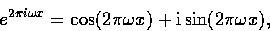we see that the Fourier transform has real and imaginary components. Moreover, the exponential form of basis function allows us to represent both real and complex valued functions by their Fourier transform.

We can show that any two basis functions of different frequencies are orthogonal by calculating their inner product and showing that it is 0. For example, for the real case and considering only the cosine terms,for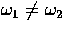, because the function being integrated is actually a cosine function itself (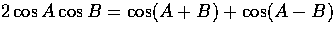) and so it has equal areas above and below the x-axis.

Thus, we project our given function f onto our basis functions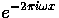to get the Fourier amplitudesfor each frequency: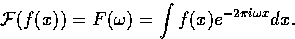In general,will be complex, say of the form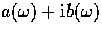.

We often express F in polar form though: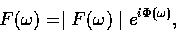whereand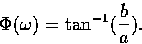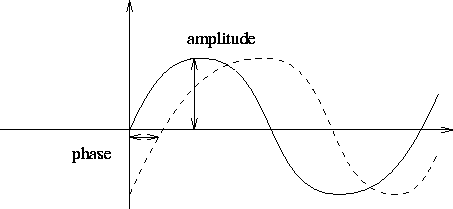The norm of the amplitude,is called the Fourier spectrum of f, and the exponent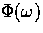is called the phase angle. The square of the amplitude is just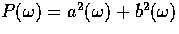and is called the power spectrum of f.

In many applications only the amplitude information is needed and the phase information is discarded. However, despite this common practice, phase information should not be ignored. In images, as in sound signals, phase carries considerable information . Oppenheim and Lim have shown that if we construct synthetic images made from the amplitude information of one image and the phase information of another, it is the image corresponding to the phase data that we perceive, if somewhat degraded.Now, having generated the Fourier transform of a function, we want to be able to reconstruct the original function from its Fourier components. This is simply done by summing up all the Fourier components multiplied by their corresponding basis function, that is,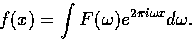This is analogous to expressing the vector v as the sum of its projections onto the basis vectors. Note that the inverse Fourier transform uses the basis functions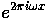, whilst the Fourier transform uses the basis functions.This prevents a sign change occurring in the reconstruction process, since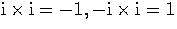.

Example Consider again the square wave form shown in the figure below.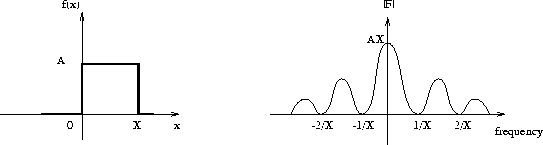Now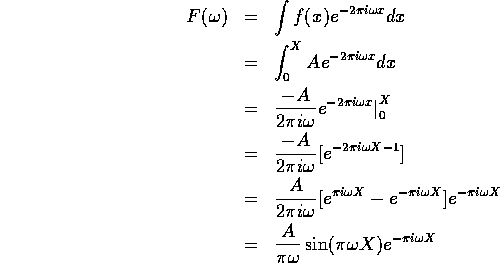This is a complex-values quantity, and the Fourier spectrum is given by its modulus,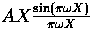.

## Important properties of the Fourier transform

Suppose we are given two functions f and g, with Fourier transforms F and G, and suppose that a and b are constants. Then

• The Fourier transform is linear, that is,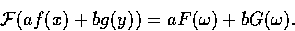• Changing spatial scale inversely affects frequency and amplitude, that is,• Shifting the function only changes the phase of the spectrum, that is,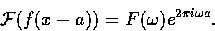We can also take advantage of symmetries in the spatial and frequency domains as follows:
• if f(x) is real, then• if f(x) is imaginary, then• if f(x) is even, then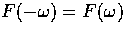• if f(x) is odd, then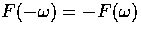.
Here, the notation * indicates the complex conjugate operation. Thus, if f(x) is real and even, thenis real and even, and if f(x) is real and odd, thenis imaginary and odd.

The Convolution Theorem tells us that convolution in the spatial domain corresponds to multiplication in the frequency domain, and vice versa. That is,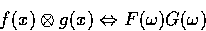and equivalently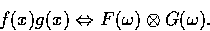Thus, convolution with large masks in the spatial domain can often be done more efficiently as multiplication in the frequency domain. Likewise, division in the frequency domain corresponds to deconvolution in the spatial domain. This is the basis by which image restoration for blur due to focus or motion is done.

## Two dimensional Fourier transforms

Now an image is thought of as a two dimensional function and so the Fourier transform of an image is a two dimensional object. Thus, if f is an image, then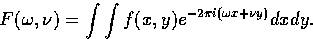Fortunately, it is possible to calculate this integral in two stages, since the 2D Fourier transform is separable. Thus, we first form the Fourier transform with respect to x: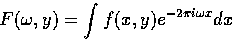and then we calculate the Fourier transform of this function of y: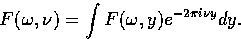Next: The discrete Fourier transform Up: Computer Vision IT412 Previous: Lecture 4
Robyn Owens
10/29/1997